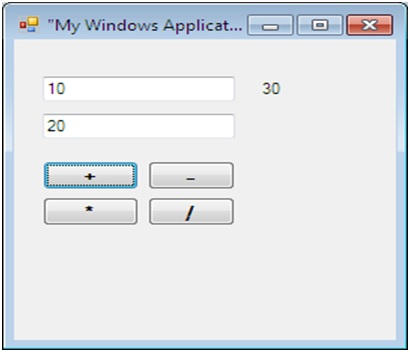# Design a Windows Application to perform Arithmetic Operations in C#

Here, we are designing a Windows Application and performing Arithmetic Operation in C#. Here, we are two TextBoxes and 4 operations to be performed: Addition, Subtraction, Multiplication and Division.
Submitted by IncludeHelp, on September 18, 2018

Here, we demonstrate basic arithmetic operations in C# Windows Application. It is very useful application to understand the basic concepts of a Windows Application in using C#.

Following operations to be performed in the given example:

2. Subtraction
3. Multiplication
4. Division

Following controls are using in the form:

• txtInput1 (TextBox) : To take first user input.
• txtInput2 (TextBox) : To take second user input.
• lblResult (Label) : To display result after performing operation.
• btnAdd (Button) : To add entered number and show result into lblResult label.
• btnSubtract (Button) : To subtract entered number and show result into lblResult label.
• btnMultiply (Button) : To multiply entered number and show result into lblResult label.
• btnDivide (Button) : To divide entered number and show result into lblResult label.

Example (Form design)C# Source Code:

```using System;
using System.Collections.Generic;
using System.ComponentModel;
using System.Data;
using System.Drawing;
using System.Linq;
using System.Text;
using System.Windows.Forms;

namespace MyWinApp
{
public partial class Form1 : Form
{
public Form1()
{
InitializeComponent();
}

private void btnAdd_Click(object sender, EventArgs e)
{
lblResult.Text = (Convert.ToInt32(txtInput1.Text) + Convert.ToInt32(txtInput2.Text)).ToString();
}

private void btnSub_Click(object sender, EventArgs e)
{
lblResult.Text = (Convert.ToInt32(txtInput1.Text) - Convert.ToInt32(txtInput2.Text)).ToString();
}

private void btnMul_Click(object sender, EventArgs e)
{
lblResult.Text = (Convert.ToInt32(txtInput1.Text) * Convert.ToInt32(txtInput2.Text)).ToString();
}

private void btnDiv_Click(object sender, EventArgs e)
{
lblResult.Text = (Convert.ToInt32(txtInput1.Text) / Convert.ToInt32(txtInput2.Text)).ToString();
}
}
}
```